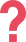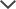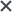If you have any questions, feel free to contact Eitan — TestPrep-Online's CAT4 expert — at ask_eitan@testprep-online.com

## About the CAT4 Level A

The Cognitive Abilities Test (or CAT4) is a cognitive test developed by GL Assessment to support schools in the understanding of students’ individual abilities and academic potential. In addition, the CAT4 is widely used as an entrance exam to many private and public schools in the UK and Ireland as well as schools around the world. The CAT4 level A exam is made according to the specifications of Year 4 in the UK, catering to children between the ages of 7 to 9.

The CAT4 level A is comprised of the following sections:

• Quantitative Reasoning-Number Series and Number Analogies
• Verbal Reasoning-Verbal Classification and Verbal Analogies
• Non-Verbal Reasoning-Figure Classification and Figure Matrices
• Spatial Ability-Figure Analysis and Figure Recognition

## CAT4 Level A Sample Questions

 CAT4 Level A Test Practice Question-Quantitative Reasoning #1 5     20     35     50    65     80     ? 75 81 85 90 95 Answer & Explanation ▼ | ▲ The correct answer is 95. In this series, every number is larger than the number before it by 15. The pattern is: +15, +15, +15, ... The last number in this series is 80, so the next number in the series should be larger than 80 by 15. 80 + 15 = 95 Therefore, 95 is the correct answer. #Note that additional practice questions for the Quantitative Reasoning battery (number analogies + number series) can be found on our CAT4 Level A practice packs.
 CAT4 Level A Test Practice Question-Verbal Reasoning #1 Pentagon→ Sides: Foot→ five fingers toes fingernails square Answer & Explanation ▼ | ▲ The correct answer is toes. A pentagon has five sides just as a foot has five toes. Five is incorrect because it does not relate to foot in the same way that sides relates to pentagon Rather, it is the relation itself for each pair, as they each have five of something. Fingers is incorrect because they are on the hand and not the foot. Fingernails is incorrect because they are on the hand and not the foot. Square is incorrect because it is related to the first pair of words (shape related). #Note that additional practice questions for the Verbal Reasoning battery (Verbal analogies + Verbal classification) can be found on our CAT4 Level A practice packs.

CAT4 Level A Test Practice Question-Non Verbal Reasoning #1The relationship between the first two figures is as follows: the second figure is the white part of the first figure, rotated 90° clockwise. The correct answer must have the same relationship with the third figure.
This means that B is the correct answer.

#Note that additional practice questions for the Non-Verbal Reasoning battery (Figure analogies + Figure classification) can be found on our CAT4 Level A practice packs.

CAT4 Level A Test Practice Question-Spatial Ability #1• First, the square was folded diagonally in half.
• Then, three holes were punched on the corners of the folded paper.
• Since the paper was folded in half once, the holes were cut through two layers of paper.
• Therefore, when the paper is unfolded it will have six holes: 3 holes x 2 layers = 6 holes.
• Since the paper was folded in half diagonally, the holes in the upper-left corner must diagonally mirror the holes in the lower-right corner.

Since answer C has three holes in the same place that they were originally punched, and three more holes that are diagonally mirroring them, equally distanced from the crease, this is the correct answer.

Answers A, B, D, and E have either more or less than six holes, so they can all be eliminated.

We are left with the 3rd choice, which is the correct answer.

#Note that additional practice questions for the Spatial Ability battery (Paper folding + Figure recognition) can be found on our CAT4 Level A practice packs.

## CAT4 Subjects

The CAT4 includes four subject-specific divisions called ‘batteries’:

Verbal Reasoning – This battery is about discovering logical connections between words. It is designed to measure your verbal comprehension, reasoning, and logic as well as your vocabulary.

Quantitative Reasoning – This battery tests one’s ability to use numbers and basic arithmetic concepts to solve simple mathematical problems. Most of the questions in this battery do not require complex calculations.

Non-verbal Reasoning – This battery tests one’s problem-solving and pattern recognition skills using various formations of shapes. These skills are needed in important school subjects such as mathematics and sciences.

Spatial Reasoning – This battery tests the ability to think and carry out tasks in three dimensions. This includes mental rotation, recognition of shapes within larger figures, and more.

## CAT4 Level A Format & Sections

CAT4 Level A is a multiple-choice exam that consists of four test batteries, each containing two short tests/sections. The following table shows the sections included in each battery:

 Verbal Reasoning Verbal ClassificationVerbal Analogies Quantitative Reasoning Number AnalogiesNumber Series Non-verbal Reasoning Figure ClassificationFigure Matrices Spatial Ability Figure AnalysisFigure Recognition

This exam is administered in three parts. The following table presents the number of questions and time allotment for each section:

 Part 1 Number of Questions Time Alloted Figure Classification 24 questions 10 minutes Figure Matrices 24 questions 10 minutes Part 2 Verbal Classification 24 questions 8 minutes Verbal Analogies 24 questions 8 minutes Number Analogies 18 questions 10 minutes Part 3 Number Series 18 questions 8 minutes Figure Analysis 18 questions 9 minutes Figure Recognition 18 questions 9 minutes

## How to Prepare for the CAT4 Level A (Year 4)

To make sure your child is adequately prepared for the CAT4, there are several things we advise you to do:

• Use our CAT practice papers for Year 4. This CAT4 test practice will provide your child with real-life simulations that reflect the real CAT4 Level A exam.
• When practicing, do not spend too much time on any one particular question. Remember that each section of the test is timed, and you should answer as many questions as you can. Try solving easier questions first so you leave more time for the harder questions.
• Take breaks when you need to during practicing, so you do not feel burnt out.
• Know your child's strengths and weaknesses through practicing so you can focus the practice on subjects that are difficult for him or her.
• When you finish a practice test, read the explanations carefully (even if you got it right!) and understand where you were wrong and why. Our practice pack provides thorough explanations and solving tips for each question.

In summary, the CAT4 Level A is a challenging test, but proper preparation increases the chances your child will ace it. Our CAT4 Level A PrepPack allows your child to familiarise him- or herself with the test and gives him or her the confidence needed to succeed.

The CAT4, CogAT, and other trademarks are the property of their respective trademark holders. None of the trademark holders are affiliated with TestPrep-Online or this website.

Need HelpNeed Help